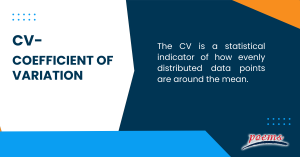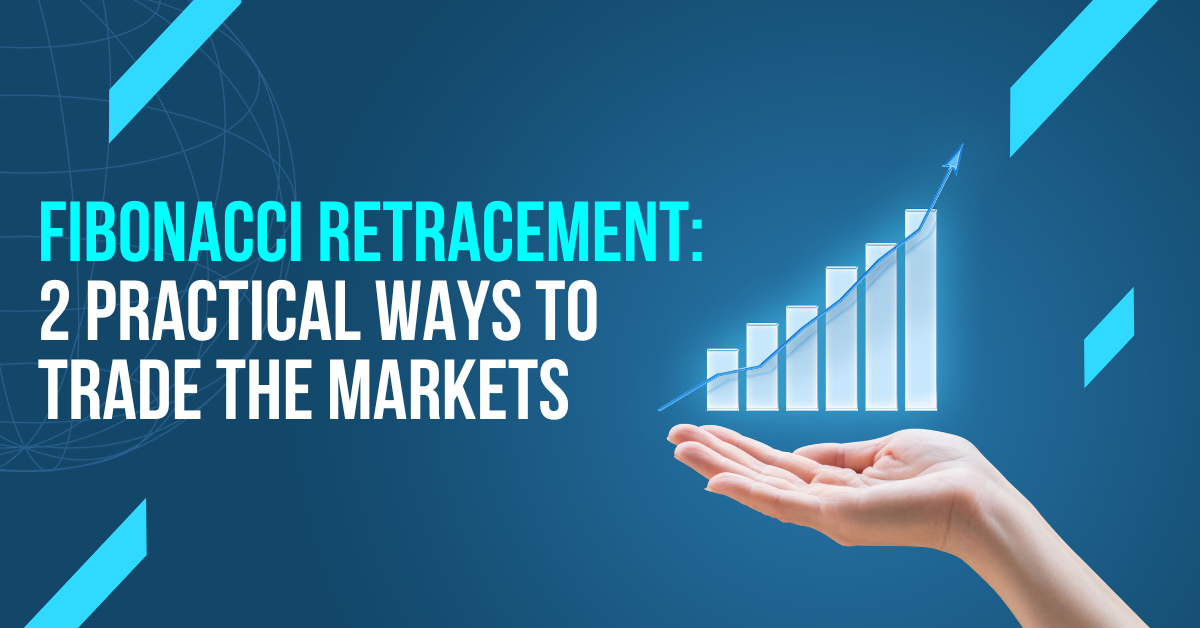# Coefficient of Variation (CV)

## Coefficient of variation

A word widely used in statistics is the coefficient of variation. In statistics, nothing should be taken at face value. The information might point to one thing, but a more thorough investigation might show something else.

Experts use a statistical metric known as the coefficient of variation to track changes in data over time. This indicator can also determine the financial risk and reward for investment operations. The coefficient of variation (CV) can be determined using a straightforward formula.

## What is CV?

The CV is a statistical indicator of how evenly distributed data points are around the mean.

The measure frequently contrasts the data dispersion between several data series. The CV offers a very straightforward and rapid method to compare several data series, in contrast to the standard deviation, which must always be viewed in the context of the data mean.

The CV is crucial when choosing investments in finance. From a financial standpoint, the financial statistic represents the risk-to-reward ratio, where the volatility reflects the danger of an investment and the mean reward.## Understanding CV

The CV demonstrates the degree of data variability in a sample compared to the population means. The CV in finance enables investors to assess how much risk or volatility is assumed relative to the return anticipated from investments.

The better the risk-return tradeoff, the lower the ratio of standard deviation to mean return should be due to the CV formula. However, for example, quartile, quintile, or decile CVs can be used to examine variance around the median or 10th percentile. They are most frequently employed to study dispersion around the mean.

Several disciplines, including chemistry, engineering, physics, economics, and neuroscience, use the CV. Economists use the risk/reward ratio to quantify economic inequality and assist in investment decision-making. It is frequently used outside of finance to check the accuracy of a certain procedure and achieve the ideal balance.

## CV formula

The CV formula computes a ratio to indicate the dispersion of your values around the mean using the standard deviation and mean of your sample data. The fundamental formula for figuring out the CV in statistical analysis is:

CV = (standard deviation/sample mean) x 100

Many experts simplify the CV formula for business purposes—like financial calculations—to apply to financial measurements like investment returns, capital gains, risk, and investment volatility. To determine the CV in terms of financial risk and reward, many analysts may also use the following formula:

CV =( volatility/expected return) x 100

The advantages of the CV are as follows:

• The CV, independent of units, depicts the link between standard deviation and mean.
• For contrasting data sets with various units or drastically differing means, the CV may be helpful. That includes when investments are chosen using the risk/reward ratio. Investors who want to take on risk could invest in assets with historically high volatility.
• Choosing the best options available becomes simple if you know how to compute the CV. This idea is helpful when a business scales up its operations because of the significant expense involved. Some of the company’s staff members could need to be let go. The CV idea aids in choosing which department should be eliminated.
• It is used to compare distributions because it is a non-dimensional number.

The disadvantages of the CV are as follows:

• The CV will approach infinity when the mean value is near zero, making it sensitive to even slight changes in the mean. It is frequently the case if the values come from a scale other than a ratio.
• Unlike the standard deviation, it is not used directly to create confidence intervals for the mean.
• When stated in its logarithmic form, calculating any variable’s CV is pointless. The reason is that the log of 1 is equal to 0. Hence, all we are doing is changing the definition of zero.
• Since CVs are independent of the number of duplicates and the confidence in the mean increases as the number of repetitions increases, they are not the optimum indicator of measurement certainty when the number of replicates changes among samples. It is advised that standard error in percent is preferable in this situation.

The range of values in a dataset is measured by the standard deviation and the CV. The standard deviation quantifies the standard deviation of the average value from the mean. The standard deviation means the coefficient of variation gauges ratio.

The CV, for instance, is employed in finance for comparing the mean expected return on an investment to the anticipated standard deviation of the investment.

The CV of observations describes the degree of variation within a population without regard to the absolute values of the data. The CV is most frequently used to evaluate a technique’s accuracy. It is also used to compare the variability of measurements taken in various units and as a metric of variability when the standard deviation is proportionate to the mean.

Example of a CV:

Consider the following data set: [80, 90, 100]. The population’s standard deviation is 8.165, and the mean is 90. The variation coefficient is 0.09. The CV is 9% as a percentage.

Generally, a CV of 20 to 30 is considered appropriate, whereas a CV of more than 30 is considered unsatisfactory.

The formula to calculate CV is:

CV = (Standard Deviation / Mean) * 100.

## Category

### Read the Latest Market Journal#### Weekly Updates 4/12/23 – 8/12/23

Published on Dec 4, 2023 27

This weekly update is designed to help you stay informed and relate economic and company...#### Fibonacci Retracement: 2 Practical Ways To Trade The Markets

Published on Nov 27, 2023 85

Overview It’s frustrating to be stopped-out of a trade, even for an experienced trader like...#### Weekly Updates 27/11/23 – 1/12/23

Published on Nov 27, 2023 34

This weekly update is designed to help you stay informed and relate economic and company...#### How Corporate Actions Affect Your Options Position

Published on Nov 23, 2023 615

Table summary Corporate Actions Δ in Option Symbol Δ in Contract Multiplier Δ in Strike...#### Weekly Updates 20/11/23 – 24/11/23

Published on Nov 20, 2023 51

This weekly update is designed to help you stay informed and relate economic and company...#### Top traded counters in October 2023

Published on Nov 16, 2023 319#### Weekly Updates 13/11/23 – 17/11/23

Published on Nov 14, 2023 47

This weekly update is designed to help you stay informed and relate economic and company...#### Weekly Updates 06/11/23 – 10/11/23

Published on Nov 6, 2023 80

This weekly update is designed to help you stay informed and relate economic and company...